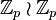# Inner automorphism group of wreath product of groups of order p

## Definition

Let$p$ be a prime number. The inner automorphism group of wreath product of groups of order p is a group obtained as follows: it is the inner automorphism group of the wreath product of groups of order p, where the acting group acts regularly: it acts by cyclic permutation of$p$ coordinates.

In other words, if$\mathbb{Z}_p$ denotes the cyclic group of order$p$, and$G = \mathbb{Z}_p \wr \mathbb{Z}_p = (\mathbb{Z}_p \times \dots \times \mathbb{Z}_p) \rtimes \mathbb{Z}_p$, then the group we want is$H = G/Z(G)$. It turns out that$Z(G)$ is precisely the diagonal subgroup of$\mathbb{Z}_p \times \dots \times \mathbb{Z}_p$.

Thus, the group$H$ that we want is a group of order$p^p$. It turns out that for$p$ odd, this group is a maximal class group (for$p = 2$, it is isomorphic to the Klein four-group). For all$p$, it has exponent p. Since its nilpotence class is$p - 1$, it is a regular p-group; however, since it has exponent$p$ and size$p^p$, it is not an absolutely regular p-group.

## Particular cases

Prime number$p$ Wreath product$\mathbb{Z}_p \wr \mathbb{Z}_p$ Group of interest Order$p^p$ Nilpotency class$p - 1$
2 dihedral group:D8 Klein four-group 4 1
3 wreath product of Z3 and Z3 unitriangular matrix group:UT(3,3) 27 2
5 wreath product of Z5 and Z5 inner automorphism group of wreath product of Z5 and Z5 3125 4

## Families

Larger collections of groups in which this group figures: